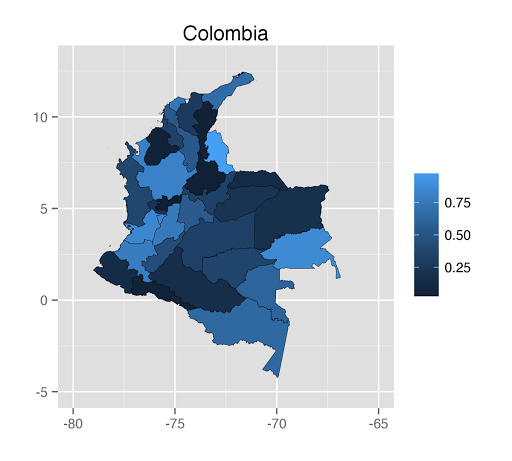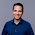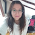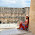## lunes, 6 de abril de 2015

### Making maps in R with ggplot

This post is devoted with the quick (and free) solution of a problem that maybe some statisticians face in their jobs. Maps are becoming a valuable tool of information and you will find that R could be a comprehensive solution for this type of task.

You will need to distinguish two types of data: the cartography data (containing the necessary information to make the map) and the variable of interest that you want to make explicit in the map. The geographic data could be downloaded for free in this webpage (http://www.diva-gis.org/gdata). For example, I just downloaded the geographic data of my beloved country: Colombia.

First, you call the proper libraries:

rm(list=ls(all=TRUE))
options(encoding = "UTF-8")

library(sp)
library(RColorBrewer)
library(ggplot2)
library(maptools)
library(scales)

Then, you read the shapes of your map. For example, Colombia is divided into departments. This shape is given by the file "COL_adm1.shp”. After that, you must merge this data with your variable of interest. For this particular case, I will generate some random senseless numbers.

setwd("~/Desktop/COL_adm")
ohsColI2 <- fortify(ohsCol2)
grupo2 <- data.frame(id = unique(ohsColI2[ , c("id")]))
grupo2[ , "Porcentaje"] <- runif(nrow(grupo2), 0, 1)
ohsColI2 <- merge(ohsColI2, grupo2, by = "id")

Later, you make the map by using the ggplot2 library. Notice that you can customize your map as you want. This is a mere example:

mapColDep <- ggplot() +
geom_polygon(data=ohsColI2, aes(x=long, y=lat, group = group,
fill = Porcentaje), colour ="black", size = 0.1) +
labs(title = "Colombia", fill = "") +
labs(x="",y="",title="Colombia") +
scale_x_continuous(limits=c(-80,-65))+
scale_y_continuous(limits=c(-5,13))
mapColDep

It’s time to save your map. Just write down these lines in your code:

ggsave(mapColDep, file = "mapColDep.png", width = 5, height = 4.5, type =
"cairo-png")

This is it. If you want to go deeper (for example, making the map by municipalities), you just use the shape: "COL_adm2.shp” and you assure that the variable of interest is properly defined. This is the final map:PD: Thanks to Daniel, Jesús and William for valuable feedback and suggestions.

#### 3 comentarios:

1.Andres, excelente post! Estaba buscando como hacer un mapa de Venezuela por estados y esto me salvo. Ahora, queria preguntarte cual archivo de los descargados en gdata tiene la tabla de codigos para saber que id number corresponde a cual estado. Saludos (va sin acentos)

2.Muchas gracias por compartir tan valiosa información!!!!!

3.Gracias! Súper útil!!!